## Semiconductors

To understand semiconductors one first has to understand the physics behind them. 0.0.1. Postulates of Quantum mechanicsUncertainty principle we can not now the position and the momentum at the same time: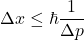When we now the particle energy we can not now the particle postion in time.Particles evolve in time like waves. And the momentum of a particle is directly realted to its wavelength.

(1)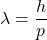Therefore electrons must be described with the wave function. The probability where the electron is at the moment can be described with0.0.2. How do we do quantum mechanicsWith the Schrödinger equations which is actually a wave equation.

(2)or

(3)and the solution of this equations turns out to beor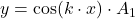or when we write in in complex form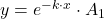0.0.3. The Electron Wavenumber kIn equation 2 and 3 we used k, because the differential equation is easier to solve with the constant k instead of the term mentioned in equation 4.

(4)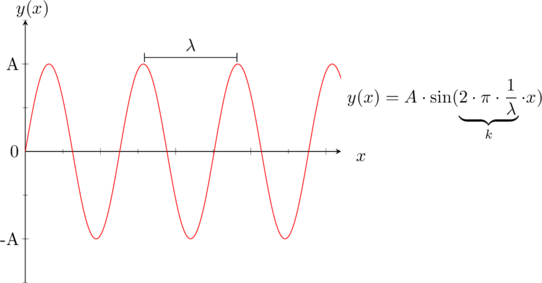In quantum mechanics,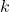does directly relate to the momentum of the particle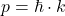. From standard physics we know equation 5

(5)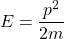When inserting equation 1 in equation 5 We get equation 6 and when we rearrange everything equation 7.

(6)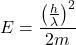(7)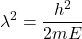Furthermore we know from the figure above that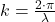When we insert this in equation 7 we get after some rearinging equation 10 which is the same as equation 4. 0.0.4. Infinite Potential WellNow we want to find a solution to the differential equation. Let’s assume we have a cube of silicon. The electrons are for sure inside this cube/lattice. So the probability that they are outside of the latice is zero. This behaviour can also be described with the graphic below: C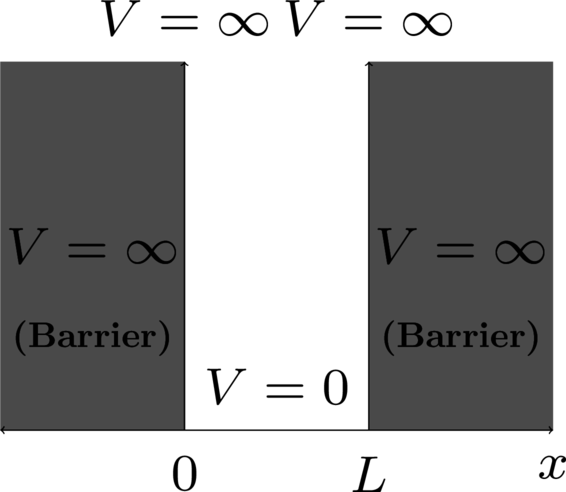} For the condition of a solution of the differential equation one can say that the probability of finding an electron must be zero at both ends, therefore the only solution would be a sine (see the possible solutions for the differential equation).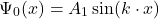Furthermore we now that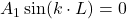therefore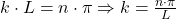. So therefore k can only take certain values, which means the energy is restricted to certain values. The last thing that remains now is to solve for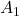. We further know equation 8, which says integral of the probability of the particle must be one.

(8)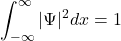Therefore we end up with the following:

(9)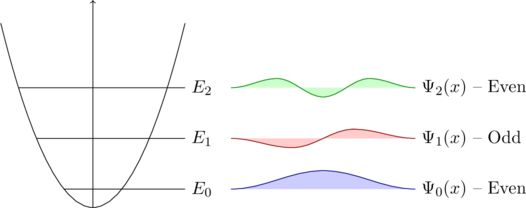(10)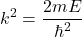(11)Now want to calculate. To do that we have to calculate the total number of states divided by the total volume of the semiconductor.

(12)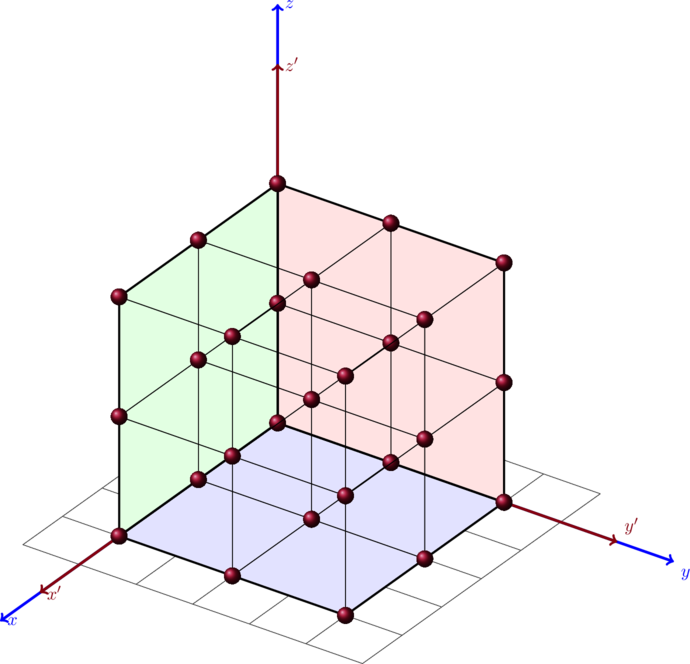Assuming that the apples are equally spaced as in the plot above one can say that eacht apple is in a distance to the other with a distance of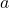. The density apple per volume is in this casewhich is one apple per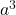. The total number of apples is know the volume divided by the apple densityThe volume of a spherical shell is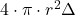. From before we know that the states of an electron are defined like the following: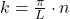therefore the states are in a distance to each other of. Futher more we can say that we have a state volume, thereforein this volume is justwhich is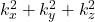and therefore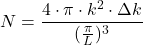When we only take the positive k_x, k_y, k_z direction we also get a factor of. The density of states in a certain volume is then given by the following formula: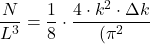Now we want to substitute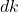with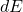. We already know: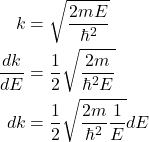With this information we can then replace k in the original equation which results in the following: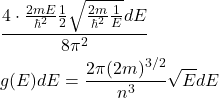And because an electron can have an up and down spin we get the following: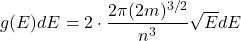or 1. intrinsic & extrinsic semiconductors Where p-type and n-type materials meet is called a p-n junction. Electrons from the n-type material move to the p-type material and close the holes and vice versa. This is called \textbf{diffusion} and leads to a {depletion layer} In an n-channel mosfet the inversion is called inversion because the p-type material looks like n-type.